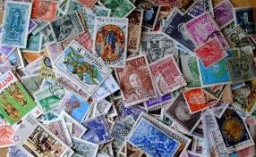# Three-euro 8145

Martin bought two-euro and three-euro stickers for 65 euros. There were five times more than two euros. How many stickers of all kinds did Martin buy?

a =  25
b =  5

### Step-by-step explanation:

65 = 2a+3b
a=5b

65 = 2·a+3·b
a=5·b

2a+3b = 65
a-5b = 0

Row 2 - 1/2 · Row 1 → Row 2
2a+3b = 65
-6.5b = -32.5

b = -32.5/-6.5 = 5
a = 65-3b/2 = 65-3 · 5/2 = 25

a = 25
b = 5

Our linear equations calculator calculates it.Did you find an error or inaccuracy? Feel free to write us. Thank you!

Tips for related online calculators
Do you have a system of equations and looking for calculator system of linear equations?